Maths-
General
Easy

Question

# Twelve students compete for a race. The number of ways in which first three places can be taken, is-

## 12 ! – 3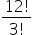12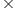1110    3 !Hint:

## The correct answer is: 121110

### The number of ways to select the winner of the first prize is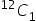The number of ways to select the winner of the second prize is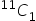(11, since one student is already given a prize)    The number of ways to select the winner of the third prize is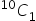(10, since two students are already given a prize)             Thus, total number of ways is××= 12 × 11 × 10 = 1320#### With Turito Foundation.#### Get an Expert Advice From Turito.